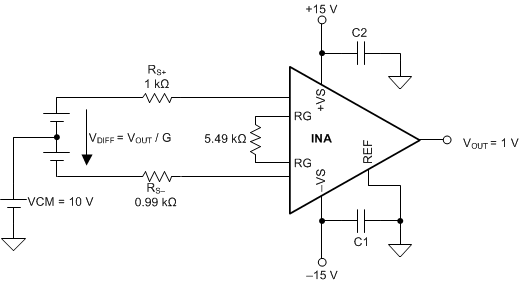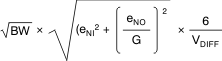SBOS893D August   2018  – June 2020

PRODUCTION DATA.

1. Features
2. Applications
3. Description
1.     Device Images
4. Revision History
5. Device Comparison Table
6. Pin Configuration and Functions
7. Specifications
8. Detailed Description
1. 8.1 Overview
2. 8.2 Functional Block Diagram
3. 8.3 Feature Description
4. 8.4 Device Functional Modes
9. Application and Implementation
1. 9.1 Application Information
2. 9.2 Typical Application
3. 9.3 Other Application Examples
10. 10Power Supply Recommendations
11. 11Layout
12. 12Device and Documentation Support
13. 13Mechanical, Packaging, and Orderable Information

• D|8
• DRG|8
• DGK|8
• DRG|8
• DGK|8

#### 8.3.6 Error Sources

Most modern signal-conditioning systems calibrate errors at room temperature. However, calibration of errors that result from a change in temperature is normally difficult and costly. Therefore, minimize these errors by choosing high-precision components, such as the INA821, that have improved specifications in critical areas that impact the precision of the overall system. Figure 64 shows an example application.Figure 64. Example Application With G = 10 V/V and a 1-V Output Voltage

Resistor-adjustable devices (such as the INA821) show the lowest gain error in G = 1 because of the inherently well-matched drift of the internal resistors of the differential amplifier. At gains greater than 1 (for instance, G = 10 V/V or G = 100 V/V), the gain error becomes a significant error source because of the contribution of the resistor drift of the 24.7-kΩ feedback resistors in conjunction with the external gain resistor. Except for very high gain applications, the gain drift is by far the largest error contributor compared to other drift errors, such as offset drift.

The INA821 offers excellent gain error over temperature for both G > 1 and G = 1 (no external gain resistor). Table 5 summarizes the major error sources in common INA applications and compares the three cases of G = 1 (no external resistor) and G = 10 (5.49-kΩ external resistor) and G = 100 (499-Ω external resistor). All calculations are assuming an output voltage of VOUT = 1 V. Thus, the input signal VDIFF (given by VDIFF= VOUT/G) exhibits smaller and smaller amplitudes with increasing gain G. In this example, VDIFF = 1 mV at G = 1000. All calculations refer the error to the input for easy comparison and system evaluation. As Table 5 shows, errors generated by the input stage (such as input offset voltage) are more dominant at higher gain, while the effects of output stage are suppressed because they are divided by the gain when referring them back to the input. the gain error and gain drift error are much more significant for gains greater than 1 because of the contribution of the resistor drift of the 24.7-kΩ feedback resistors in conjunction with the external gain resistor. In most applications, static errors (absolute accuracy errors) can readily be removed during calibration in production, while the drift errors are the key factors limiting overall system performance.

### Table 4. System Specifications for Error Calculation

QUANTITY VALUE UNIT
VOUT 1 V
VCM 10 V
VS 1 V
RS+ 1000 Ω
RS– 999 Ω
RG tolerance 0.01 %
RG drift 10 ppm/°C
Temperature range upper limit 105 °C

### Table 5. Error Calculation

ERROR SOURCE ERROR CALCULATION INA821 VALUES
SPECIFICATION UNIT G = 1 ERROR (ppm) G = 100 ERROR (ppm) G = 1000 ERROR (ppm)
ABSOLUTE ACCURACY AT 25°C
Input offset voltage VOSI / VDIFF 35 µV 35 350 3500
Output offset voltage VOSO / (G × VDIFF) 300 µV 350 350 350
Input offset current IOS × maximum (RS+, RS–) / VDIFF 0.5 nA 1 5 50
CMRR (min) VCM / (10CMRR/20 × VDIFF) 92 (G = 1),
112 (G = 10),
132 (G = 100)
dB 251 251 251
PSRR (min) (VCC – VS)/ (10PSRR/20 × VDIFF) 110 (G = 1),
114 (G = 10),
130 (G = 100)
dB 3 20 32
Gain error from INA (max) GE(%) × 104 0.02 (G = 1),
0.15 (G = 10, 100)
% 200 1500 1500
Gain error from external resistor RG (max) GE(%) × 104 0.01 % 100 100 100
Total absolute accuracy error (ppm) at 25°C, worst case sum of all errors 940 2576 5738
Total absolute accuracy error (ppm) at 25°C, average rms sum of all errors 487 1603 3834
DRIFT TO 105°C
Gain drift from INA (max) GTC × (TA – 25) 5 (G = 1),
35 (G = 10, 100)
ppm/°C 400 2800 2800
Gain drift from external resistor RG (max) GTC × (TA – 25) 10 ppm/°C 800 800 800
Input offset voltage drift (max) (VOSI_TC / VDIFF) × (TA – 25) 0.4 µV/°C 32 320 3200
Output offset voltage drift [VOSO_TC / ( G × VDIFF)] × (TA – 25) 5 µV/°C 400 400 400
Offset current drift IOS_TC × maximum (RS+, RS–) ×
(TA – 25) / VDIFF
20 pA/°C 2 16 160
Total drift error to 105°C (ppm), worst case sum of all errors 1634 4336 7360
Total drift error to 105°C (ppm), typical rms sum of all errors 980 2957 4348
RESOLUTION
Gain nonlinearity 10 (G = 1, 10),
15 (G = 100)
ppm of FS 10 10 15
Voltage noise (at 1 kHz)eNI = 7,
eNO = 65
µVPP 1335 886 3566
Current noise (at 1kHz) IN × maximum (RS+, RS–) × √BW / VDIFF 0.13 pA/√Hz 0.4 2 11
Total resolution error (ppm), worst case sum of all errors 1345 896 3581
Total resolution error (ppm), typical rms sum of all errors 1335 886 3566
TOTAL ERROR
Total error (ppm), worst case sum of all errors 3919 7808 16724
Total error (ppm), typical rms sum of all errors 1726 3478 6806